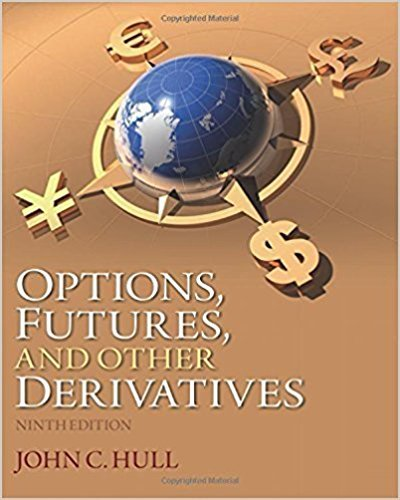×
×

# Solutions for Chapter 19: The Greek Letters## Full solutions for Options, Futures, and Other Derivatives | 9th Edition

ISBN: 9780133456318Solutions for Chapter 19: The Greek Letters

Solutions for Chapter 19
4 5 0 365 Reviews
20
4
##### ISBN: 9780133456318

This textbook survival guide was created for the textbook: Options, Futures, and Other Derivatives, edition: 9. Chapter 19: The Greek Letters includes 30 full step-by-step solutions. This expansive textbook survival guide covers the following chapters and their solutions. Since 30 problems in chapter 19: The Greek Letters have been answered, more than 15215 students have viewed full step-by-step solutions from this chapter. Options, Futures, and Other Derivatives was written by and is associated to the ISBN: 9780133456318.

Key Business Terms and definitions covered in this textbook

the tendency for the mix of unobserved attributes to become undesirable from the standpoint of an uninformed party

• circular-flow diagram

a visual model of the economy that shows how dollars flow through markets among households and firms

• consumption

spending by households on goods and services, with the exception of purchases of new housing

• cross-price elasticity of demand

a measure of how much the quantity demanded of one good responds to a change in the price of another good, computed as the percentage change in quantity demanded of the first good divided by the percentage change in price of the second good

• efficient scale

the quantity of output that minimizes average total cost

• equality

the property of distributing economic prosperity uniformly among the members of society

• fixed costs

costs that do not vary with the quantity of output produced

• in-kind transfers

transfers to the poor given in the form of goods and services rather than cash

• marginal revenue

the change in total revenue from an additional unit sold

• market economy

an economy that allocates resources through the decentralized decisions of many firms and households as they interact in markets for goods and services

• mutual fund

an institution that sells shares to the public and uses the proceeds to buy a portfolio of stocks and bonds

• permanent income

a person’s normal income

• political economy

the study of government using the analytic methods of economics

• price elasticity of demand

a measure of how much the quantity demanded of a good responds to a change in the price of that good, computed as the percentage change in quantity demanded divided by the percentage change in price

• price elasticity of demand

a measure of how much the quantity demanded of a good responds to a change in the price of that good, computed as the percentage change in quantity demanded divided by the percentage change in price

• progressive tax

a tax for which highincome taxpayers pay a larger fraction of their income than do low-income taxpayers

• random walk

the path of a variable whose changes are impossible to predict

• scarcity

the limited nature of society’s resources

• substitutes

two goods for which an increase in the price of one leads to an increase in the demand for the other

• substitution effect

the change in consumption that results when a price change moves the consumer along a given indifference curve to a point with a new marginal rate of substitution

×